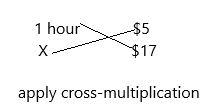# Texas Go Math Grade 4 Lesson 18.4 Answer Key Budget a Weekly Allowance

Refer to our Texas Go Math Grade 4 Answer Key Pdf to score good marks in the exams. Test yourself by practicing the problems from Texas Go Math Grade 4 Lesson 18.4 Answer Key Budget a Weekly Allowance.

## Texas Go Math Grade 4 Lesson 18.4 Answer Key Budget a Weekly Allowance

Essential Question

How can you budget a weekly allowance?
Weekly allowance budgeting is just what it sounds like: Individuals give themselves a set amount of money to spend each week. This method differs from traditional budgeting, which tends to look at finances and spending allowances by month. Weekly allowances are typically for discretionary expenses, like dining out or shopping. Fixed expenses, also referred to sometimes as mandatory expenses, play a part in determining a weekly allowance but aren’t included in it. To create a weekly allowance, start big with your monthly budget. Categorize your expenses by mandatory expenses, like rent, car payments, insurance, student loan payments, utility bills and groceries. After accounting for your monthly recurring obligations—don’t forget about savings!—you can use the remaining money to cover your variable expenses.

Remember: Expenses can be fixed or variable

Unlock the Problem

Luis receives an allowance of $15 each week. He wants to make a budget to show how he will use his allowance. In his budget, the expenses should not be more than his allowance. A budget is an organized plan for spending and saving money. Help Luis budget his weekly allowance. Find the weekly expenses. Luis’ weekly expenses are listed in the table below. Complete the table. How much will Luis save for charity?Answer: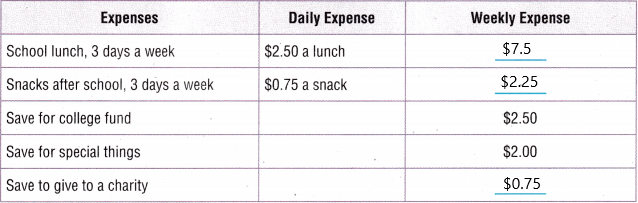Explanation: The amount Luis receives each week is$15.
Her expenses were given in the above diagram. So we need to find out weekly expenses.
School lunch for 3 days a week=$2.50*3=$7.5
Snacks for 3 days aweek=$0.75*3=$2.25
College fund=$2.50 Special things=$2.00
The total amount she spends on above  expenses=7.5+2.25+2.50+2.00=14.25
For charity=weekly allowances-above 4 expenses
=$15-$14.25
Charity =$0.75 Find the total for each weekly expense. Lunch Expense:$_______ + $_____ +$_____ = $_____ a week Snack Expense:$ _______ $_______ +$ _______ = $_______ a week Total expenses before charity expense:$ _______ + $_______ +$_______ + $_______ =$ _______
To find the amount Luis will donate to charity, _______ the total expenses from his weekly allowance.
Charity Expense: $_______ –$ 14.25 = $_______ So, Luis will save$ _______ per week to give to charity.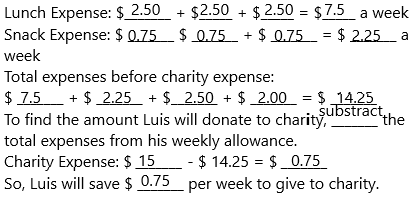Math Talk

Mathematical Processes
When might Luis change his budget? Explain.
1. Comparing his budget to actual spending: Identify how much of the original budget has been spent, and how much remains. If you’re using a good project management tool you should be able to figure this out pretty quickly.  This information gives you a reality check of where you stand versus budget.  And don’t forget: Focus on the big variances from the budget; don’t sweat the small stuff.
2. Determine how the change order will affect the cost: Project change orders often come with an updated budget—so you could be facing more money allocated or less. Either way, the project manager’s approach doesn’t differ greatly here (except maybe in the stress department). .

Share and Show

Since Carver goes to a school that is not near his house, he receives an allowance of $50 each week. He wants to make a budget to show how he will use his allowance. For each of 5 days, he pays$3.40 to ride the city bus, $3.60 for lunch, and$1 for snacks. He wants to save half of what money he has left each week. How much money will Carver save each week?

Complete the table.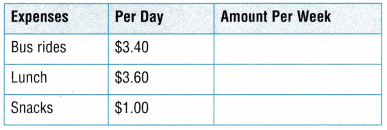Find the total for each expense for the week.
Bus rides: $_________ +$ _________ + $_________ +$ _________ + $_________ =$ _________
Lunch: $_________ +$ _________ + $_________ +$ _________ + $_________ =$ _________
Snacks: $_________ a day × _________ days =$ _________
Total expenses each week: $_________ +$ _________ + $_________ =$ _________
Difference between allowance and expenses: $50 –$ _________ = $_________ Half of the money Carver has left after expenses:$ _________ ÷ 2 = $_________ So, Carver will save$ _________ each week.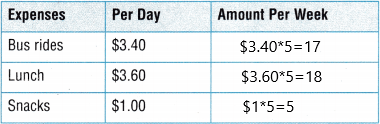Half of the money Carver has left after expenses: $10÷2=$5.
So, the carver will save $5 each week. Use the information given to answer the question. Question 2. Elijah receives a weekly allowance of$25. How could he budget his allowance among spending, saving, including college, and sharing? Complete the table.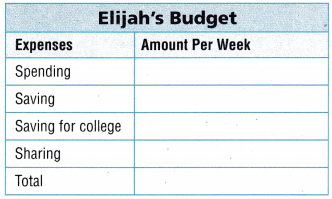Explanation:
Given weekly allowances:$25 He used to budget on the given expenses those are spending, saving, saving for college, sharing. (4 expenses). Assume if he saves$6.25 on every expense then total $25. Question 3. Lea gets a weekly allowance. She saves$14 a week from her allowance. Each week, she puts aside $2 for charity, saves$4 for college, and $12 for fun. What is Leaps weekly allowance? Answer:$32 is her weekly allowance.
Explanation:
The amount she saves from weekly allowance=$14 The amount she saves for charity=$2
The amount she saves for college is $4 The amount she saves for fun=$12
The Leaps weekly allowances are $14+$2+$4+$12=$32. Problem Solving Question 4.H.O.T. Multi-Step Stacy gets$15 a week allowance. She is saving for a bike that costs $321. Each week she gives$3 to charity, spends $4, and puts$5 in her college fund. If she saves the rest of her allowance for her bike, how many weeks will it take her to save enough money to buy her bike?
The amount Stacy gets a weekly allowance=$15 The amount she wants to save for the bike=$321
The amount she spends on charity, spending, college fund every week=$3+$4+$5=$12
Now extra $3 she needs to save for the bike. The number of weeks she wants to save$3 to buy a bike=$321/$3=107.

Question 5.
Reasoning Pero gets a weekly allowance of $10 for doing chores and$8 per hour babysitting his brother. His goal is to save $10 a week for college, give$10 a week to charity, and have $14 a week to spend. He babysits the same amount each week. How many hours does he need to babysit each week to reach his goal? Explain. Answer: 3 hours. The amount he gets a weekly allowance for chores=$10
The amount he gets for babysitting=$8 per hour He wants to save for college, charity, and his spendings=$10+$10+$14=$34. If he saves for college$10 of his chores allowance then the left $24 need to save from the babysitting allowance. The number of hours he needs to sit each week to reach his goal is ‘X’. For 1 hour –$8
X     –   $24 ?Now this can be written as: 8X=$24*1
8X=$24 X=24/8 X=3 hours. Go Math Answer Key 4th Grade Lesson 18.4 Question 6. H.O.T. Multi-Step Henry pays$10 per week for a gym membership, $10 a week for his cell phone, and saves$15 each week. He earns $10 for every lawn he mows. What is the least number of lawns that he needs to mow each week to cover his budgeted expenses? Explain. Answer: 4 lawns. The amount Henry pays for gym membership=$10
The amount he pays for cell phone=$10 The amount he saves every week=$15
The total amount he saves for expenses=$10+$10+$15=$35
The amount he gets if he mows each lawn=$10 The least number of lawns he needs to mow each week is ‘X’ for 1 lawn he gets$10
X lawn he gets $35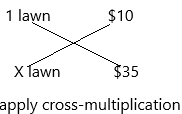10X=35 X=35/10 X=3.5 (at least three and a half(3 1/2)lawn he has to mow). He should do 3 lawns completely and on another lawn, he has to mow half the lawn. But If he does 4 lawns then he will get 4*$10=$40. Approximately 4 lawns he has to mow. Daily Assessment Task Fill in the bubble completely to show your answer. Question 7. Sammy is saving$5 out of his $10 allowance each week for summer science camp. He must divide the rest between his savings account, a charity of his choice, and his college fund. Sammy gives$1 per week to a local charity. He puts the same amount in his savings account as his college fund. How much does Sammy put in his college fund each week?
(A) $2.00 (B)$2.50
(C) $4.50 (D)$4.00Explanation:
The total allowance he gets every week=$10 The amount he saves for summer science each week=$5
Now he has left with $5 (10-5=5). From this$5 he was saving for charity each week=$1 The amount he saves for the college funds is$5-$1=$4. (To get college fund savings he did a subtraction that is he subtracted left allowance and charity savings then he will get the college fund savings).

Question 8.
Lisa wants to buy a tablet computer that costs $300. She gets a weekly allowance of$12. If she saves $5 each week toward the cost of the tablet computer, how many weeks will it take her to save enough to buy it? (A) 300 weeks (B) 20 weeks (C) 60 weeks (D) 30 weeks Answer: Option C is correct.The cost of the tablet computer=$300
The allowance she gets weekly=$12 If she saves$5 each week then how many weeks she needs to save to buy a tablet computer? To calculate $300/5=60. Therefore, she needs to save$5, in 60 weeks.

Multi-Step Juan is having a party to earn money for his local animal shelter. His goal is to give the shelter $250. He charges each person$5 to attend the party, and 35 people attend. If Juan saves $5 of his allowance each week for the animal shelter, how long will it take him to save the rest of the$250 for the shelter?
(A) 7 weeks
(B) 75 weeks
(C) 5 weeks
(D) 15 weeksExplanation:
The amount he wants to give for shelter=$250 The amount he charged from the people who attended the party was$5
The number of people who attended the party=35
If he is saving $5 each week then how many weeks will take to complete his savings that are$250. But he already got $175 after the party. In$250 he earned $175. Now we have to calculate for an extra$75.
($250-$175=$75). The time is taken by him to save$75=$75/5=15 weeks. TEXAS Test Prep Question 10. Each week, Carol saves$24, pays $56 for her expenses, and gives$8 to the charity of her choice. If she makes $8 per hour, how many hours must she work to make enough money to meet this budget? (A) 3 hours (B) 7 hours (C) 11 hours (D) 10 hours Answer: Option C is correct.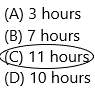Explanation: The amount she saves every week on her expenses, charity, savings=$24+$56+$8=$88 If she works$8 per hour, then the number of hours she needs to work to make enough money to meet his budget is $88/8=11 hours. ### Texas Go Math Grade 4 Lesson 18.4 Homework and Practice Answer Key Use the information given to answer the question. Question 1. Carlos has a weekly allowance of$24. He spends $4 on snacks and$6 on entertainment each week. He is saving the rest for a printer that costs $224. How many weeks will it take Carlos to save enough money to buy the printer? Answer: 16 weeks. Explanation: The allowance Carlos gets every week is$24
The amount he spends on her snacks and entertainment=$4+$6=$10 The cost of the printer=$224
The rest of the amount is $24-$10=$14. The number of weeks will it take Carlos to save enough money to buy the printer=$224/14=16 weeks.
Carlos takes 16 weeks to save money.
Question 2.
Brenda’s weekly allowance is $15. She puts aside$5 of her weekly allowance for gifts and gives $2 to charity. She divides what’s left into equal amounts for spending money and music downloads. How much spending money does Brenda have each week? Answer:$4 for spending money and $4 for music downloads.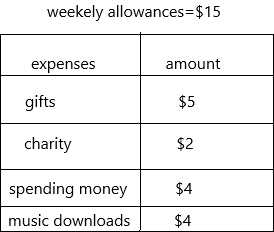Explanation: The weekly allowance of Brenda’s is$15
The amount she put aside for gifts and charity=$5+$2=$7. The amount left was$15-$7=$8.
She divides what’s left into equal amounts for spending money and music downloads=8/2=4.
$4 for spending amount and$4 for music downloads.
The amount for spending money is $4. Problem Solving Question 3. Darleen gets$10 a week allowance. She walks her neighbor’s dog 6 days each week and earns $3 each day. She earns$7 an hour doing odd jobs. Darleen wants to save $15 a week, give$5 a week to charity, and have at least $20 each week to spend. How many hours of odd jobs does she need to do each week to earn enough money? Explain. Answer: 5 hours in a week. The allowance Darleen gets every week=$10
The amount she gets on dog walking=6*$3=$18 (6 days and $3). The amount she earns on an odd job=$7 per hour.
The total amount she earns each week=$10+$18=$28 The amount she spends on savings, charity, and her weekly spendings is$15+$5+$20=$40. (40-28=12). She wants to find it for$12.
The number of hours of odd jobs does she need to do each week to earn enough money=’X’.
For 1 hour she earns $7 and X hours she earns$12. By applying cross-multiplication.7X=12
X=12/7
X=2 hours.

If Darleen wants to increase her spending money to $25 a week and earn an extra$12 a week for a phone plan, how many extra hours a week will she need to work odd jobs? Explain.
Answer: 18 hours in a week.
The allowance Darleen gets every week=$10 The amount she gets on dog walking=6*$3=$18 (6 days and$3).
The amount she earns on an odd job=$7 per hour. The amount she earns for a phone plan=$12
The total amount she earns each week=$10+$18+$12=$40
The amount she increased on spending up to $25. In the above question, her spendings are$20 but now she increased so her total spendings are $20+$25=$45. The amount she spends on savings, charity, and her weekly spendings=$15+$5+$45=$65. The number of hours she needs to odd jobs to earn enough money be’X’. This can be calculated by subtracting the total weekly allowance and savings each week=$65-$40=25. Now we have to calculate for$25.
for 1 hour she spends $7 and for X hours she spends$25.7X=25
X=25/7
X=3.5 approximately 4 hours.

Lesson Check

Question 5.
Jim gets a weekly allowance. He keeps $8 for spending money, saves$5 and gives $2 to charity. How much is Jim’s weekly allowance? (A)$15
(B) $13 (C)$11
(D) $16 Answer: Option A is correct.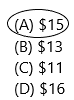Explanation: The weekly allowance of Jim is ‘X’. The amount he put aside for spending money, savings, charity=$8+$5+$2=$15. The weekly allowance of Jim is$15.

Question 6.
Gianna budgets her allowance so she uses equal amounts for spending, saving, and gifts. Her weekly allowance is $13.50. How much does Gianna save each week? (A)$6.75
(B) $4.25 (C)$5.00
(D) $4.50 Answer: Option D is correct.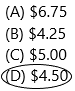Explanation: The allowance Gianna gets each week=$13.50
She divides it equally for her spending, saving, and gifts.
The amount she saves each week is $13.50/3=$4.5

Question 7.
Multi-Step Lily gets $13 a week allowance. She saves$3, gives $2 a week to the soup kitchen, and divides the rest between spending money and clog treats. How much money does Lily spend each week on dog treats? (A)$8
(B) $7 (C)$5
(D) $4 Answer: Option D is correct.Explanation: The allowance Lily gets each week=$13
The amount she puts aside for her savings and the soup kitchen=$3+$2=$5 The rest of the amount is$13-$5=$8. This $8 is divided into half for spending money and for dog treats. After dividing$8 means 8/2=4.
$4 for money spending and the left$4 for dog treats.
Here in the question asked was only for dog treats. That’s why the answer is $4. Question 8. Multi-Step Hunter is sang for a cordless drill. The drill costs$160. Hunter gets a weekly allowance of $15. He keeps$8 a week for spending money and saves the rest. How many weeks will it take him to save enough money to buy the drill?
(A) 23 weeks
(B) 20 weeks
(C) 11 weeks
(D) 15 weeks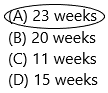Explanation:
The cost of drill=$160 The allowance he gets each week=$15
The amount he put aside for spending money=$8 The amount he saves the rest=$15-$8=$7.
The number of weeks will it take him to save enough money to buy the drill=’X’. this can be explained below.
For 1 week, he saves $7 and for X number of weeks, he is saving$160. we need to find out X. By applying cross-multiplication:$7X=$160
X=160/7
X=23.

Question 9.
Multi-Step Wendy gets a weekly allowance of $8. She keeps$5 for spending money and divides the rest evenly into savings and giving to charity. how much does Wendy give to charity each week?
(A) $2.00 (B)$1.50
(C) $1.75 (D)$1.25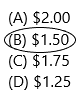Explanation:
The allowance Wendy gets weekly=$8 The amount she put aside for spending money was$5.
The rest of the amount is $8-$5=$3. This$3 is divided into half for savings and charity. This $3 is divided as$3/2=$1.5$1.5 is for savings and the other $1.5 is for charity. Here the question asked was how much amount she gives to charity. She gives$1.5 to charity.

Multi-Step Dylan gets a weekly allowance of $10. He also earns$5 an hour gardening for his aunt. He wants to have $12 a week for spending money and to save at least$15 a week to buy a new guitar. How many hours will he need to work in his aunt’s garden each week to reach his goal?
(A) 3 hours
(B) 2 hours
(C) 4 hours
(D) 5 hours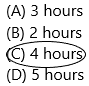The allowance Dylan gets weekly=$10 The amount he gets for gardening =$5 per hour.
The amount he put aside for his spending and savings for his guitar=$12+$15=$27. The time he wants to find$27-$10=$17.
1 hour, the aunt pays $5. For X hours, the aunt pays$17.